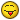###Author Topic: Quick favor I could do with :p  (Read 2622 times)

####spike43884##### Quick favor I could do with :p
« on: August 09, 2016, 06:46:25 AM »
I was just stopping by because I need 2 mathematical equations (preferably as simple as possible).
Equation 1, needs to be able to check if a position is a specific magnitude away from another position in a 2 dimensional space.
Equation 2 needs to do the same as equation 1, except in a 3 dimensional space.

I have absolutely no idea what equations to useAutism can allow so much joy, and at the same time sadness to be seen. Our world is weird, and full of contradiction everywhere, yet somehow at moments seems to come together, and make near perfect sense.

####Numsgil##### Re: Quick favor I could do with :p
« Reply #1 on: August 09, 2016, 01:46:31 PM »
eq1: (x1 - x2)^2 + (y1 - y2)^2 <= distance^2

For positions (x1, y1) and (x2, y2).  It's basically just checking if x2 is within the circle of radius distance centered at x1.

eq2: (x1 - x2)^2 + (y1 - y2)^2 + (z1 - z2)^2 <= distance^2

For positions (x1, y1, z1) and (x2, y2, z2).  Same deal, but now it's a sphere.

This extends in to higher dimensions.  You just keep adding in squared terms.

####spike43884##### Re: Quick favor I could do with :p
« Reply #2 on: August 10, 2016, 11:07:56 AM »
eq1: (x1 - x2)^2 + (y1 - y2)^2 <= distance^2

For positions (x1, y1) and (x2, y2).  It's basically just checking if x2 is within the circle of radius distance centered at x1.

eq2: (x1 - x2)^2 + (y1 - y2)^2 + (z1 - z2)^2 <= distance^2

For positions (x1, y1, z1) and (x2, y2, z2).  Same deal, but now it's a sphere.

This extends in to higher dimensions.  You just keep adding in squared terms.

Thanks.
Autism can allow so much joy, and at the same time sadness to be seen. Our world is weird, and full of contradiction everywhere, yet somehow at moments seems to come together, and make near perfect sense.